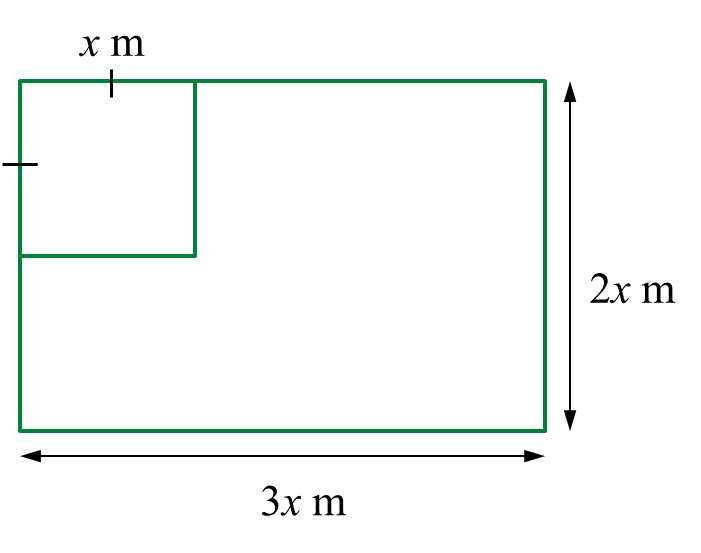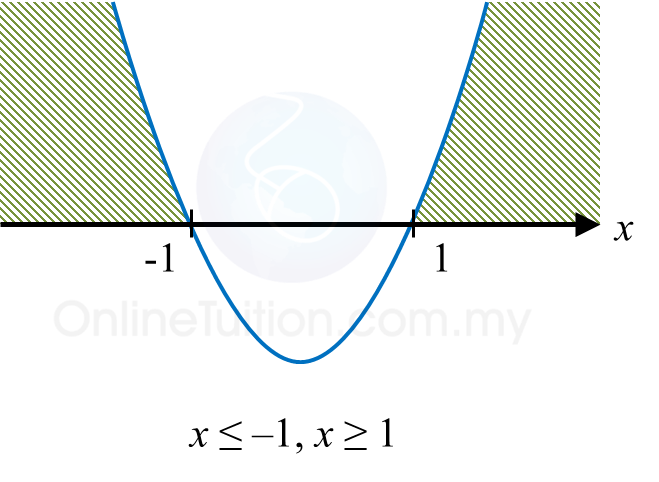# SPM Additional Mathematics 2018, Paper 1 (Question 17 – 19)

Question 17 (4 marks):

Solution:
(a)
a > 0, a ≠ 1

(b)
$\begin{array}{l}{\mathrm{log}}_{3}y=\frac{2}{{\mathrm{log}}_{xy}3}\\ \frac{{\mathrm{log}}_{xy}y}{{\mathrm{log}}_{xy}3}=\frac{2}{{\mathrm{log}}_{xy}3}\\ {\mathrm{log}}_{xy}y=2\\ y={\left(xy\right)}^{2}\\ y={x}^{2}{y}^{2}\\ \frac{1}{{x}^{2}}=\frac{{y}^{2}}{y}\\ y=\frac{1}{{x}^{2}}\end{array}$

Question 18 (3 marks):
Diagram 8 shows the graph y = a (xp)2 + q, where a, p and q are constants. The straight line y = –8 is the tangent to the curve at point H.Diagram 8

(a) State the coordinates of H.
(b) Find the value of a.

Solution:
(a)

(b)

Question 19 (3 marks):
Faizal has a rectangular plywood with a dimension 3x metre in length and 2x metre in width. He cuts part of the plywood into a square shape with sides of x metre to make a table surface.
Find the range of values of x if the remaining area of the plywood is at least (x2 + 4) metre2.

Solution:Area of plywood – area of square ≥ (x2 + 4)
3x(2x) – x2x2 + 4
6x2x2x2 ≥ 4
4x2 ≥ 4
x2 – 1 ≥ 0
(x + 1)(x – 1) ≥ 0
x ≤ –1 or x ≥ 1
Thus, x ≥ 1 (length is > 0)